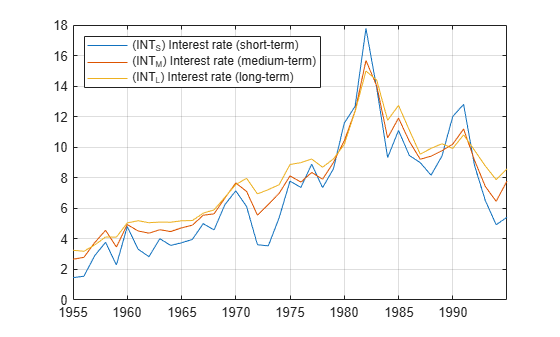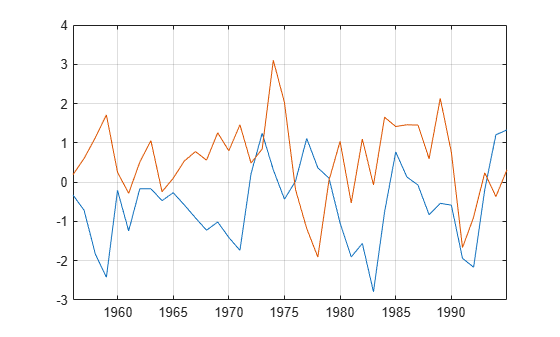# jcitest

Johansen cointegration test

## Syntax

```[h,pValue,stat,cValue,mles] = jcitest(Y) [h,pValue,stat,cValue,mles] = jcitest(Y,Name,Value) ```

## Description

Johansen tests assess the null hypothesis H(r) of cointegration rank less than or equal to r among the `numDims`-dimensional time series in `Y` against alternatives H(`numDims`) (`trace` test) or H(r+1) (`maxeig` test). The tests also produce maximum likelihood estimates of the parameters in a vector error-correction (VEC) model of the cointegrated series.

```[h,pValue,stat,cValue,mles] = jcitest(Y)``` performs the Johansen cointegration test on a data matrix `Y`.

```[h,pValue,stat,cValue,mles] = jcitest(Y,Name,Value)``` performs the Johansen cointegration test on a data matrix `Y` with additional options specified by one or more `Name,Value` pair arguments.

## Input Arguments

 `Y` `numObs`-by-`numDims` matrix representing `numObs` observations of a `numDims`-dimensional time series yt, with the last observation the most recent. Y cannot have more than 12 columns. Observations containing `NaN` values are removed. Initial values for lagged variables in VEC model estimation are taken from the beginning of the data.

### Name-Value Pair Arguments

Specify optional comma-separated pairs of `Name,Value` arguments. `Name` is the argument name and `Value` is the corresponding value. `Name` must appear inside quotes. You can specify several name and value pair arguments in any order as `Name1,Value1,...,NameN,ValueN`.

`'model'`

Character vector, such as `'H2'`, or cell vector of character vectors specifying the form of the deterministic components of the VEC(q) model of yt:

`$\Delta {y}_{t}=C{y}_{t-1}+{B}_{1}\Delta {y}_{t-1}+\dots +{B}_{q}\Delta {y}_{t-q}+DX+{\epsilon }_{t}$`

If r < `numDims` is the cointegration rank, then C = AB, where A is a `numDims`-by-r matrix of error-correction speeds and B is a `numDims`-by-r matrix of basis vectors for the space of cointegrating relations. X contains any exogenous terms representing deterministic trends in the data. For maximum likelihood estimation, it is assumed that εt  ~ NID(0,Q), where Q is the innovations covariance matrix.

Values of `model` are those considered by Johansen .

ValueForm of Cyt−1 + DX
`'H2'`

AB´yt−1. There are no intercepts or trends in the cointegrated series and there are no deterministic trends in the levels of the data.

`'H1*'`

A(B´yt−1+c0). There are intercepts in the cointegrated series and there are no deterministic trends in the levels of the data.

`'H1'`

A(B´yt−1+c0)+c1. There are intercepts in the cointegrated series and there are deterministic linear trends in the levels of the data. This is the default value.

`'H*'`A(B´yt−1+c0+d0t)+c1. There are intercepts and linear trends in the cointegrated series and there are deterministic linear trends in the levels of the data.
`'H'`A(B´yt−1+c0+d0t)+c1+d1t. There are intercepts and linear trends in the cointegrated series and there are deterministic quadratic trends in the levels of the data.

Deterministic terms outside of the cointegrating relations, c1 and d1, are identified by projecting constant and linear regression coefficients, respectively, onto the orthogonal complement of A.

`'lags'`

Scalar or vector of nonnegative integers indicating the number q of lagged differences in the VEC(q) model of yt.

Lagging and differencing a time series reduce the sample size. Absent any presample values, if yt is defined for t = 1:N, then the lagged series ytk is defined for t = k + 1:N. Differencing reduces the time base to k+2:N. With q lagged differences, the common time base is q+2:N and the effective sample size is T = N−(q+1).

Default: 0

`'test'`

Character vector, such as `'trace'`, or cell vector of character vectors indicating the type of test to be performed. Values are `'trace'` or `'maxeig'`. The default value is `'trace'`. Both tests assess the null hypothesis H(r) of cointegration rank less than or equal to r. Statistics are computed using the effective sample size T and ordered estimates of the eigenvalues of C = AB′, λ1 > ... > λd, where d = `numDims`.

• When the value is `'trace'`, the alternative hypothesis is H(`numDims`). Statistics are:

`$-T\left[\mathrm{log}\left(1-{\lambda }_{r+1}\right)+\dots +\mathrm{log}\left(1-{\lambda }_{numDims}\right)\right]$`

• When the value is `'maxeig'`, the alternative hypothesis is H(r+1). Statistics are:

`$-T\mathrm{log}\left(1-{\lambda }_{r+1}\right)$`

`'alpha'`

Scalar or vector of nominal significance levels for the tests. Values must be between 0.001 and 0.999.

Default: 0.05

`'display'`

Character vector, such as `'off'`, or cell vector of character vectors indicating whether or not to display a summary of test results and parameter estimates in the Command Window.

ValueDisplay
`'off'`No display to the command window. This is the default if `jcitest` is called with only one output argument (`h`).
`'summary'`Display a summary of test results. Null ranks r = 0:`numDims` − 1 are displayed in the first column of each summary. Multiple tests are displayed in separate summaries. This is the default if `jcitest` is called with more than one output argument (that is, if `pValue` is computed), and is unavailable if `jcitest` is called with only one output argument (`h`).
`'params'`Display maximum likelihood estimates of the parameter values associated with the reduced-rank VEC(q) model of yt. This display is only available if `jcitest` is called with five output arguments (that is, if `mles` is computed). Displayed parameter values are returned in `mles.rn`(`m`).`paramVals` for null rank r = `n` and test `m`.
`'full'`Display both `summary` and `params`.

Character vectors values are expanded to the length of any vector value (the number of tests). Vector values must have equal length.

## Output Arguments

`h`

`numTests`-by-`numDims` tabular array of Boolean decisions for the tests.

Rows of `h` correspond to tests specified by the input arguments, and the software labels the rows `t1`,`t2`,...,`tu`, where `u` = `numTests`. Variables of `h` correspond to different, maintained cointegration ranks r = 0,...,`numDims` – 1, and the software labels the variables `r0`,`r1`,...,`rR`, where `R` = `numDims` – 1. To access results stored in `h`, for example, the result for test `m` of null rank `n`, use `h.rn(m)`.

Values of `h` equal to `1` (`true`) indicate rejection of the null of cointegration rank r in favor of the alternative. Values of `h` equal to `0` (`false`) indicate a failure to reject the null.

`pValue`

`numTests`-by-`numDims` tabular array of right-tail probabilities of the test statistics.

Rows of `pValue` correspond to tests specified by the input arguments, and the software labels the rows `t1`,`t2`,...,`tu`, where `u` = `numTests`. Variables of `pValue` correspond to different, maintained cointegration ranks r = 0,...,`numDims` – 1, and the software labels the variables `r0`,`r1`,...,`rR`, where `R` = `numDims` – 1. To access results stored in `pValue`, for example, the result for test `m` of null rank `n`, use `pValue.rn(m)`.

`stat`

`numTests`-by-`numDims` tabular array of test statistics, determined by the `test` name-value pair argument.

Rows of `stat` correspond to tests specified by the input arguments, and the software labels the rows `t1`,`t2`,...,`tu`, where `u` = `numTests`. Variables of `stat` correspond to different, maintained cointegration ranks r = 0,...,`numDims` – 1, and the software labels the variables `r0`,`r1`,...,`rR`, where `R` = `numDims` – 1. To access results stored in `stat`, for example, the result for test `m` of null rank `n`, use `stat.rn(m)`.

`cValue`

`numTests`-by-`numDims` tabular array of critical values for right-tail probabilities, determined by the `alpha` name-value pair argument. `jcitest` loads tables of critical values from the file `Data_JCITest.mat`, then linearly interpolates test-critical values from the tables. Tabulated values were computed using methods described in .

Rows of `cValue` correspond to tests specified by the input arguments, and the software labels the rows `t1`,`t2`,...,`tu`, where `u` = `numTests`. Variables of `cValue` correspond to different, maintained cointegration ranks r = 0,...,`numDims` – 1, and the software labels the variables `r0`,`r1`,...,`rR`, where `R` = `numDims` – 1. To access results stored in `cValue`, for example, the result for test `m` of null rank `n`, use `cValue.rn(m)`.

`mles`

`numTests`-by-`numDims` tabular array of structures of maximum likelihood estimates associated with the VEC(q) model of yt. Each structure contains these fields.

FieldDescription
`paramNames`

Cell vector of parameter names, of the form:

{`A``B``B1`,...,`Bq``c0``d0``c1``d1`}

Elements depend on the values of `lags` and `model`.

`paramVals`Structure of parameter estimates with field names corresponding to the parameter names in `paramNames`.
`res` T-by-`numDims` matrix of residuals, where T is the effective sample size, obtained by fitting the VEC(q) model of yt to the input data.
`EstCov`Estimated covariance Q of the innovations process εt.
`eigVal`Eigenvalue associated with H(r).
`eigVec`Eigenvector associated with the eigenvalue in `eigVal`. Eigenvectors v are normalized so that vS11v = 1, where S11 is defined as in .
`rLL` Restricted loglikelihood of `Y` under the null.
`uLL`Unrestricted loglikelihood of `Y` under the alternative.

Rows of `mles` correspond to tests specified by the input arguments, and the software labels the rows `t1`,`t2`,...,`tu`, where `u` = `numTests`. Variables of `mles` correspond to different, maintained cointegration ranks r = 0,...,`numDims` – 1, and the software labels the variables `r0`,`r1`,...,`rR`, where `R` = `numDims` – 1. To access results stored in `mles`, for example, the result for test `m` of null rank `n`, use `mles.rn(m)`. You can further access the fields of the structure using dot notation, for example, enter `mles.rn(m).paramNames` for the parameter names.

## Examples

collapse all

```load Data_Canada Y = Data(:,3:end); names = series(3:end); plot(dates,Y) legend(names,'location','NW') grid on```Test for cointegration:

`[h,pValue,stat,cValue,mles] = jcitest(Y,'model','H1');`
```************************ Results Summary (Test 1) Data: Y Effective sample size: 40 Model: H1 Lags: 0 Statistic: trace Significance level: 0.05 r h stat cValue pValue eigVal ---------------------------------------- 0 1 37.6886 29.7976 0.0050 0.4101 1 1 16.5770 15.4948 0.0343 0.2842 2 0 3.2003 3.8415 0.0737 0.0769 ```
`h,pValue`
```h=1×3 table r0 r1 r2 _____ _____ _____ t1 true true false ```
```pValue=1×3 table r0 r1 r2 _________ ________ ________ t1 0.0050497 0.034294 0.073661 ```

Plot estimated cointegrating relations ${B}^{\prime }{y}_{t-1}+{c}_{0}$:

```YLag = Y(2:end,:); T = size(YLag,1); B = mles.r2.paramVals.B; c0 = mles.r2.paramVals.c0; plot(dates(2:end),YLag*B+repmat(c0',T,1)) grid on```## Algorithms

• If `jcitest` fails to reject the null of cointegration rank r = 0, the inference is that the error-correction coefficient C is zero, and the VEC(q) model reduces to a standard VAR(q) model in first differences. If `jcitest` rejects all cointegration ranks r less than `numDims`, the inference is that C has full rank, and yt is stationary in levels.

• The parameters A and B in the reduced-rank VEC(q) model are not uniquely identified, though their product C = AB is. `jcitest` constructs `B` = `V`(:,1:r) using the orthonormal eigenvectors `V` returned by `eig`, then renormalizes so that ```V'*S11*V = I```, as in .

• To test linear constraints on the error-correction speeds A and the space of cointegrating relations spanned by B, use `jcontest`.

• Time series in `Y` might be stationary in levels or first differences (i.e., I(0) or I(1)). Rather than pretesting series for unit roots (using, e.g., `adftest`, `pptest`, `kpsstest`, or `lmctest`), the Johansen procedure formulates the question within the model. An I(0) series is associated with a standard unit vector in the space of cointegrating relations, and its presence can be tested using `jcontest`.

• To convert VEC(q) model parameters in the `mles` output to VAR(q+1) model parameters, use `vec2var`.

• Deterministic cointegration, where cointegrating relations, perhaps with an intercept, produce stationary series, is the traditional sense of cointegration introduced by Engle and Granger  (see `egcitest`). Stochastic cointegration, where cointegrating relations produce trend-stationary series (that is, `d0` is nonzero), extends the definition of cointegration to accommodate a greater variety of economic series.

• Unless higher-order trends are actually present in the data, models with fewer restrictions can produce good in-sample fits, but poor out-of-sample forecasts.

## References

 Engle, R. F. and C. W. J. Granger. "Co-Integration and Error-Correction: Representation, Estimation, and Testing." Econometrica. v. 55, 1987, pp. 251–276.

 Hamilton, J. D. Time Series Analysis. Princeton, NJ: Princeton University Press, 1994.

 Johansen, S. Likelihood-Based Inference in Cointegrated Vector Autoregressive Models. Oxford: Oxford University Press, 1995.

 MacKinnon, J. G., A. A. Haug, and L. Michelis. “Numerical Distribution Functions of Likelihood Ratio Tests for Cointegration.” Journal of Applied Econometrics. v. 14, 1999, pp. 563–577.

 Turner, P. M. “Testing for Cointegration Using the Johansen Approach: Are We Using the Correct Critical Values?” Journal of Applied Econometrics. v. 24, 2009, pp. 825–831.